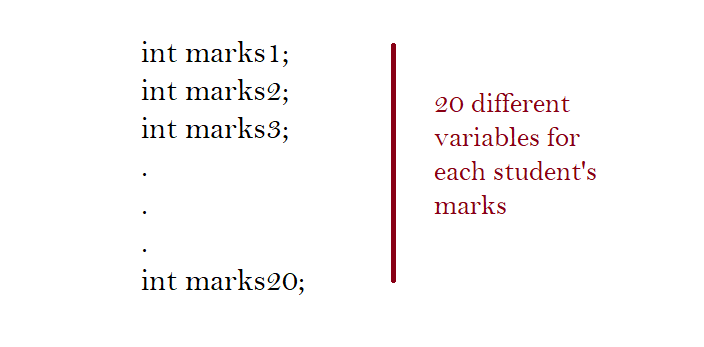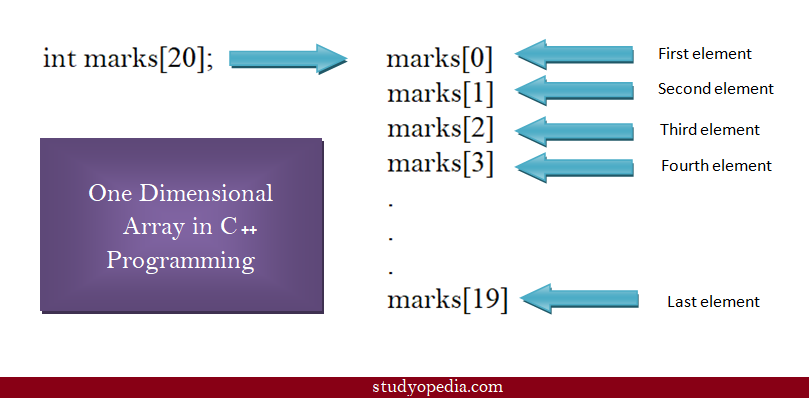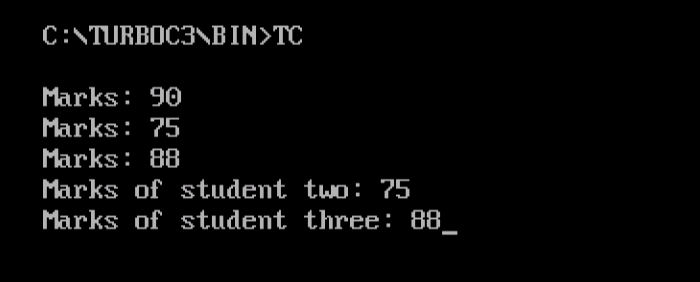# Arrays in C++ Programming

## 12 Jan Arrays in C++ Programming

Array is a collection of elements, of fixed size and type, for example, collection of 10 elements of type int. It is a data structure to group elements. In this lesson, we will learn how to work with arrays in C++ Language.

Let’s see another example to understand the concept.

A class has 20 students and you want to arrange the marks of all the 20 students. You can do this using the following two methods,

Method 1
Declare 20 different variables for each student’s marks, with its own identifier. That would be cumbersome, right?Method 2
Declare a single array variable and store the 20 different values (marks) in contiguous memory locations i.e. use one-dimensional arrays.

You saw two methods above. Definitely, Method 2 is better, since you do not need to declare different variables for the same type of elements.

Accordingly, method 2 would look like the following,Above you saw how arrays work in C++ Programming. For individual array elements, we use the index under square brackets. Index 0 is for first element, Index 1 is for the second element and it goes on. This means first element have index 0, and the last element is 19 for our 20 elements array for students’ marks. These terms would be new to you since you just begin learning arrays.

Now, we will get into more details about array declaration and initialization.

## How to declare arrays in C++

For array declaration in C++ Programming, you need to do what you did in C Programming. Specify the datatype and the count of elements you want the array to be. Here, we are discussing about one-dimensional arrays. For example, write , if you want to declare an array with 3 elements.

The following syntax will make the concept clearer,

Here,
datatype: C++ data type such as int, float, etc.
name_of_array: The name of an array (variable)
size_of_array: The size of array under square brackets. Add value greater than zero.

The following example shows the perfect way of declaring arrays in C++,

Above, we declared an array, with datatype int, name of array marks, with 3 elements, since the size we specified is 3. The variable array marks now holds 3 elements.

## How to initialize arrays

To initialize arrays, you need to add the array values in a single line. Continuing the previous example,

To initialize the above, add the array values like this in braces,

Above, we added marks for 3 students by initializing our array. Always remember, element one would come at index 0 and the last element at size – 1. For example,

## First C++ Program with Arrays

To move further, let us see a complete C++ Program, and learn how to declare and initialize arrays. We will also see how to access array elements,

The following is the output, displaying declaration, initialization of one-dimensional array and how to access individual array elements,In this lesson we learned how to work with Arrays in C++ Programming. Now, we will learn how to implement Multi-Dimensional Arrays in C++.[email protected]

We work to create free tutorials for all.